International
Tables for
Crystallography
Volume B
Reciprocal space
Edited by U. Shmueli

International Tables for Crystallography (2006). Vol. B. ch. 1.3, pp. 42-43   | 1 | 2 |

## Section 1.3.2.6.7. The Poisson summation formula

G. Bricognea

aMRC Laboratory of Molecular Biology, Hills Road, Cambridge CB2 2QH, England, and LURE, Bâtiment 209D, Université Paris-Sud, 91405 Orsay, France

#### 1.3.2.6.7. The Poisson summation formula

| top | pdf |

Let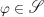, so that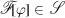. Let R be the lattice distribution associated to lattice Λ, with period matrix A, and let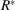be associated to the reciprocal lattice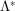. Then we may write: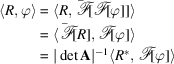i.e.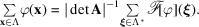This identity, which also holds for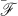, is called the Poisson summation formula. Its usefulness follows from the fact that the speed of decrease at infinity of ϕ and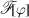are inversely related (Section 1.3.2.4.4.3), so that if one of the series (say, the left-hand side) is slowly convergent, the other (say, the right-hand side) will be rapidly convergent. This procedure has been used by Ewald (1921)[see also Bertaut (1952), Born & Huang (1954)] to evaluate lattice sums (Madelung constants) involved in the calculation of the internal electrostatic energy of crystals (see Chapter 3.4in this volume on convergence acceleration techniques for crystallographic lattice sums).

When ϕ is a multivariate Gaussian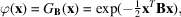then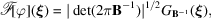and Poisson's summation formula for a lattice with period matrix A reads: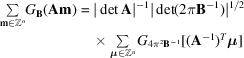or equivalently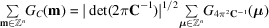with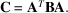### ReferencesBertaut, E. F. (1952). L'énergie électrostatique de réseaux ioniques. J. Phys. Radium, 13, 499–505.Google ScholarBorn, M. & Huang, K. (1954). Dynamical theory of crystal lattices. Oxford University Press.Google ScholarEwald, P. P. (1921). Die Berechnung optischer und electrostatischer Gitterpotentiale. Ann. Phys. Leipzig, 64, 253–287.Google Scholar